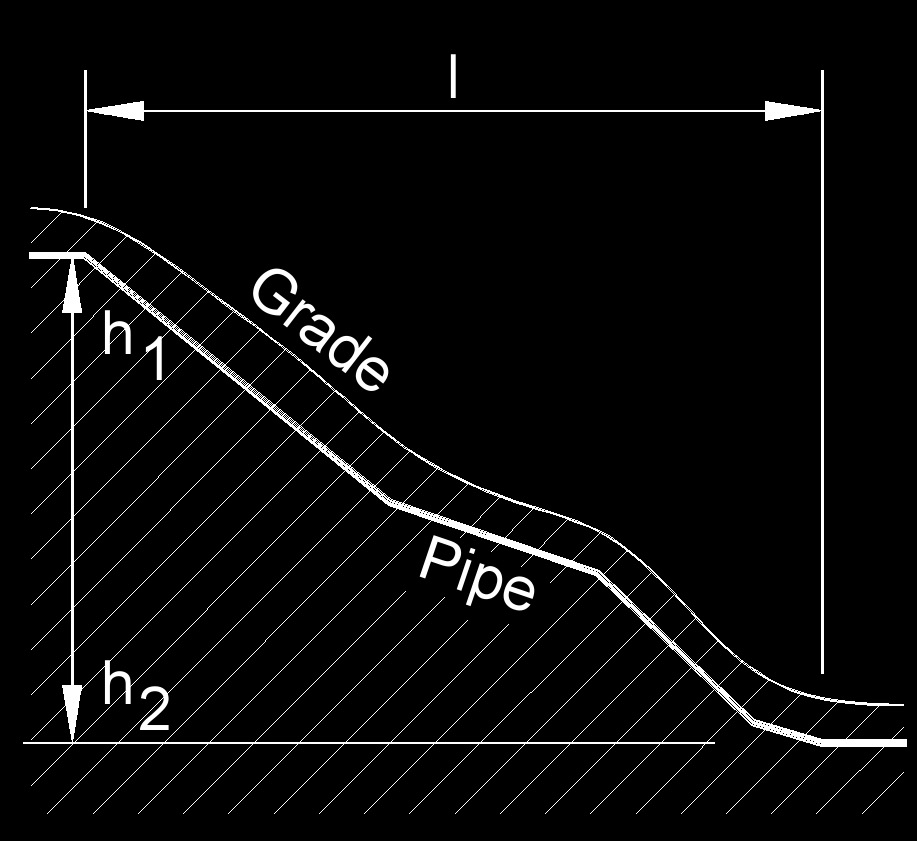Written by Jerry Ratzlaff on . Posted in Dimensionless NumbersHydraulic gradient, abbreviated as i, is a dimensionless number expressing the change in height (pressure) to length between any two points.

$$\large{ i = \frac { h_1 \;-\; h_2} { l} }$$

$$\large{ i = \frac{ Q }{ k \; A_c } }$$

$$\large{ i = \frac{v}{k} }$$     (Darcy velocity)

Where:

$$\large{ i }$$ = hydraulic gradient

$$\large{ A_c }$$ = area cross-section of flow

$$\large{ v }$$ = Darcy velocity or flux

$$\large{ Q }$$ = flow rate

$$\large{ k }$$ = hydraulic conductivity

$$\large{ l }$$ = length of column

$$\large{ h_1 }$$ = pressure head at point 1

$$\large{ h_2 }$$ = pressure head at point 2

Solve for:

$$\large{ h_1 = i \; l \;+\; h_2 }$$

$$\large{ h_2 = h_1 \;-\; i \; l }$$